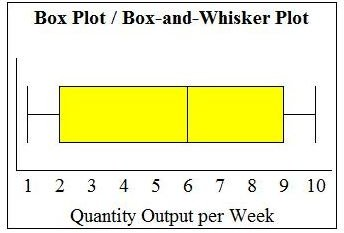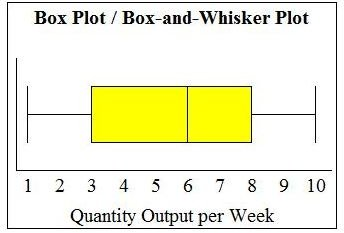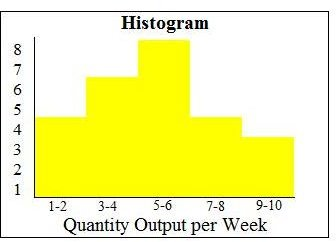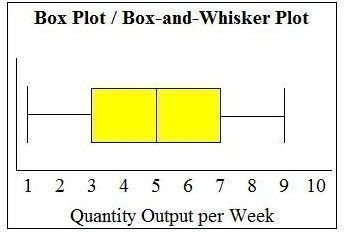# Comparing Box Plots and Histograms – Which Is the Better Tool?

Page content

## Charts Aids to Identify Variation

The goal of Six Sigma is to improve the quality and productivity of a project team or company. In order to accomplish this goal, Six Sigma uses different chart aids to identify variation among data samples. When a histogram or box plot is used to graphically represent data, a project manager or leader can visually identify where variation exists, which is necessary to identify and control causes of variation in process improvements.

What Is a Histogram?

A histogram is a type of bar chart that graphically displays the frequencies of a data set. Similar to a bar chart, a histogram plots the frequency, or raw count, on the Y-axis (vertical) and the variable being measured on the X-axis (horizontal).

The only difference between a histogram and a bar chart is that a histogram displays frequencies for a group of data, rather than an individual data point; therefore, no spaces are present between the bars. Typically, a histogram groups data into small chunks (four to eight values per bar on the horizontal axis), unless the range of data is so great that it easier to identify general distribution trends with larger groupings.

What Is a Box Plot?

A box plot, also called a box-and-whisker plot, is a chart that graphically represents the five most important descriptive values for a data set. These values include the minimum value, the first quartile, the median, the third quartile, and the maximum value. When graphing this five-number summary, only the horizontal axis displays values. Within the quadrant, a vertical line is placed above each of the summary numbers. A box is drawn around the middle three lines (first quartile, median, and third quartile) and two lines are drawn from the box’s edges to the two endpoints (minimum and maximum).

## Comparing Histograms and Box Plots

Although histograms and box plots are collectively part of the chart aid category, they do represent very different types of charts. Both charts effectively represent different data sets; however, in certain situations, one chart may be superior to the other in achieving the goal of identifying variances among data. The type of chart aid chosen depends on the type of data collected, rough analysis of data trends, and project goals.A histogram is highly useful when wide variances exist among the observed frequencies for a particular data set. As seen in the two graphs to the left, the histogram shows that there are three peaks within the data, indicating it is tri-modal (three commonly recurring groups of numbers). This is important because to improve processes, it is critical to understand what is causing these three modes. Had this data simply been graphed using a box plot, the values would average one another out, causing the distribution to look roughly normal.Another instance when a histogram is preferable over a box plot is when there is very little variance among the observed frequencies. The histogram displayed to the right shows that there is little variance across the groups of data; however, when the same data points are graphed on a box plot, the distribution looks roughly normal with a high portion of the values falling below six.The final set of graphs shows how a box plot can be more useful than a histogram. This occurs when there is moderate variation among the observed frequencies, which causes the histogram to look ragged and non-symmetrical due to the way the data is grouped. This may lead one to assume the data is slightly skewed. However, when a box plot is used to graph the same data points, the chart indicates a perfect normal distribution.